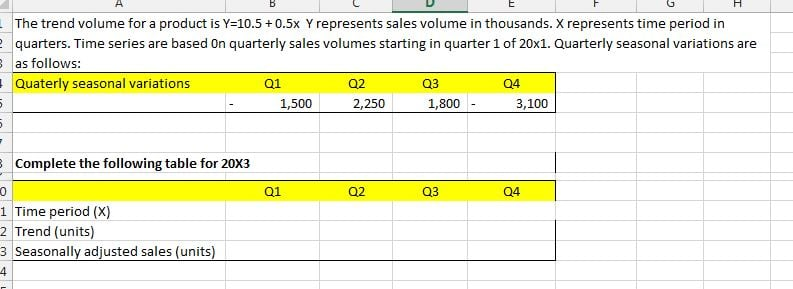Current updates regarding coronavirus (Covid-19) and the precautions AAT are taking will be continually updated on the below page.

We hope you are all safe and well and if you need us we will be here. 💚

# Y = a + bx for calculating seasonally adjusted sales

Hi All,

I came across a calculation which I could not resolve. Perhaps I was not looking from a correct angle. Can someone help me with it? I have attached a picture of the question.• I will start and perhaps someone will add their thoughts on this.

1. I have converted formula to Y = 10,500 + 500x since seasonal variances are in thousands.
2. I have worked out x=9 for 20X3 Q1 (based on 20X1 Q1 = 1)
3. Trend volume for 20X3: Q1 = 15,000, Q2 = 15,500, Q3 = 16,000, Q4 = 16,500
4. No clue afterwards.. What should go to "Time period(x) in Q1 to Q4? I can work out actual data based on trend + variance. But what on earth is this Time period(x)?
• Maybe Time period(x) is 9 for Q1, 10 for Q2, 11 for Q3 and 12 for Q4.
Then Trend (units) Q1=15,000, Q2=15,500, Q3=16,000, Q4=16,500
Then Seasonally adjusted sales Q1=14,000, Q2=17,750, Q3=17,800, Q4=13,400
Perhaps I've just answered my own question...
• Do you know what the answer should be?
• andrew96 said:

Do you know what the answer should be?

Not 100% sure if my assumptions on the latest reply were correct. I do not see any other logical possibility to resolve this.
• Well-Known FarehamRegistered Posts: 247
JJ1 said:

Maybe Time period(x) is 9 for Q1, 10 for Q2, 11 for Q3 and 12 for Q4.
Then Trend (units) Q1=15,000, Q2=15,500, Q3=16,000, Q4=16,500
Then Seasonally adjusted sales Q1=14,000, Q2=17,750, Q3=17,800, Q4=13,400
Perhaps I've just answered my own question...

This looks correct to me.
AAT
Level 2 - 2011
Level 3 - 2012
Level 4 - 2013

ACCA
F4 - Corporate Law - Dec 2015 (passed)
F5 - Performance Management - Dec 2014 (passed)
F6 - Taxation - Dec 2013 (passed)
F7 - Financial Reporting - Jun 2014 (passed)
F8 - Audit & Assurance - Dec 2015 (passed)
F9 - Financial Management - Jun 2015 (passed)